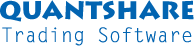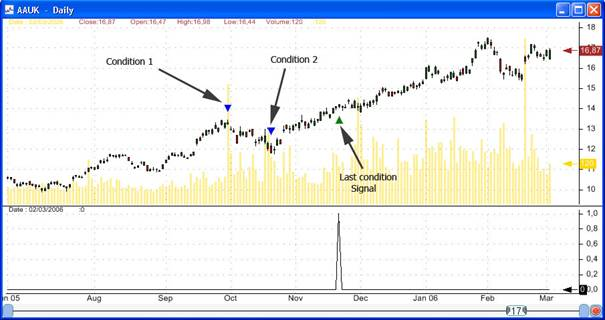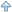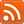#twitter-follow { border: 0;position: fixed; top: 240px; left:0;} #live-support { border: 0;position: fixed; top: 370px; left:0;} #knowledge-base { border: 0;position: fixed; top: 170px; left:0;}

1.1. Searchfor

Used to detect any complex pattern

Let you create unrestrictive rules

Syntax:

Searchfor syntax1 then syntax1 for (rule) set (special_rule)

syntax1: special_rule after period within period all

special_rule: Special rule (Supports QuantShare functions + special functions)
rule: Normal rule (Any rule that could be created using the QuantShare language)

Explanation: this function looks for successive events.
Example: search for a stock that rises 20 percent within 30 bars, then drops by 10 percent for the next 10  20 bars.

After (optional): specify that special_rule will be checked only after the number of bars specified after the keyword after

Within (optional):  specify that special_rule  must be TRUE in at least one of the bars starting from current bar + after value to current bar + after value + within value

All (optional): specify that special_rule  must be TRUE for all the bars starting from current bar + after value to current bar + after value + within value

For (optional): calculates the pattern only when the rule provided in (for) keyword is TRUE

Set (optional): The Searchfor function returns 1 on bars where the pattern is found, if we specify a formula in (set) keyword, the Searchfor function will returns the result of this function instead of 1

Special functions (that could be used in special_rule):

·         _perf (Array): Gets the percent of change from the specified array, from the bar where the previous condition occurred to the current bar.

·         _perf(Array, lag, length): Gets the percent of change from the specified array, from "the current bar minus lag minus length" to "the current bar minus lag".

·         _change (Array): Gets the raw change from the specified array, from the bar where the previous condition occurred to the current bar.

·         _change (Array, lag, length): Gets the raw change from the specified array, from "the current bar minus lag minus length" to "the current bar minus lag".

·         _avg (Array): Gets the average value of the specified array, from the bar where the previous condition occurred to the current bar.

·         _avg(Array, lag, length): Gets the average value of the specified array, from "the current bar minus lag minus length" to "the current bar minus lag".

·         _min (Array): Gets the minimum value of the specified array, from the bar where the previous condition occurred to the current bar.

·         _min (Array, lag, length): Gets the minimum value of the specified array, from "the current bar minus lag minus length" to "the current bar minus lag".

·         _max (Array): Gets the maximum value of the specified array, from the bar where the previous condition occurred to the current bar.

·         _max(Array, lag, length): Gets the maximum value of the specified array, from "the current bar minus lag minus length" to "the current bar minus lag".

·         _sd (Array): Gets the standard deviation value of the specified array, from the bar where the previous condition occurred to the current bar.

·         _sd (Array, lag, length): Gets the standard deviation value of the specified array, from "the current bar minus lag minus length" to "the current bar minus lag".

·        _ref (Array , index_lag): Gets a value from the specified array on the bar where the condition "last condition index minus the specified index_lag" occurred.

Index equals to 0 means get a value from the specified array on the bar where the last condition occurred.

Index equals to 1 means get a value from the specified array on the bar where the next to last condition occurred.

FRef (Array): same as the last function but uses zero as "index_lag"

·         _dis(index_lag): Gets the distance (in bars) between the current bar and the bar where condition "last condition index minus the specified index_lag" occurred.

Index equals to 0 means get the number of bars between the current bar and the bar where the last condition occurred.

·         _iff (condition, if_true, if_false): Evaluates and returns the result of the if_true formula if the condition is TRUE, otherwise evaluates and returns the result of theif_false formula.

·         _slope (Array): Gets the slope of the line that starts at the array value for the bar where the previous condition occurred to the array value of the current bar.

·         _slope (Array, lag, length): Gets the slope of the line that starts at the array value for "the current bar minus lag minus length" to the array value of "the current bar minus lag".

·         _aboveline (Array1, Array2): Returns TRUE if all the elements of the Array2 between the bar where the previous condition occurred to the current bar are ABOVE the line that starts at the Array1 value for the bar where the previous condition occurred to the Array1 value of the current bar.

·         _aboveline (Array1, Array2, lag, length): Returns TRUE if all the elements of the Array2 between"the current bar minus lag minus length" and "the current bar minus lag" are ABOVE the line that starts at the Array1 value for "the current bar minus lag minus length" to the Array1 value of "the current bar minus lag".

·         _belowline (Array1, Array2): Returns TRUE if all the elements of the Array2 between the bar where the previous condition occurred to the current bar are BELOW the line that starts at the Array1 value for the bar where the previous condition occurred to the Array1 value of the current bar.

·         _belowline (Array1, Array2, lag, length): Returns TRUE if all the elements of the Array2 between"the current bar minus lag minus length" and "the current bar minus lag" are BELOW the line that starts at the Array1 value for "the current bar minus lag minus length" to the Array1 value of "the current bar minus lag".

Notes:

_change(close) is equivalent to _change(close, 0, _dis(0))

Example:

Let us say we want to look for stocks that have the following pattern:

·         Positive performance of at least 20 percent in the last 30 bars

·         Then a negative performance of at least -10 percent in a period between 10 and 50 bars, during this period the stock close price must be lower than the price at which the last condition occurred

·         Then a positive performance of at least 20 percent within the next 30 bars

Here is the formula that describes this pattern:

rule = Searchfor perf(close, 30) > 20

then

_perf(close) < -10 && _max(close) <= _ref(close)

after 10 within 50

then

_perf(close) > 20

within 30;

Explanation:

·         Positive performance of at least 20 percent in the last 30 bars. "Perf" is a vector-based function, while "_perf" is a special function.

perf(close, 30) > 20

·         Then a negative performance of at least -10 percent in a period between 10 and 50 bars, the _perf functions doesnt need a period parameter as perf do, because it will use the (current bar - last condition bar) as the period parameter

_perf(close) < -10

during this period, the stock close price must be lower than the price at which the last condition occurred.
Note the difference between close and _ref(close). Close references the close price for the current bar, while _ref(close) references the close price of the last condition bar. _ref(close, 1) references the close price of the next to last condition bar if it exists (otherwise it takes the last condition bar). Now, we only have one condition (perf(close, 30) > 20), because the current condition is not evaluated yet.

_max(close) <= _ref(close)

·         Then a positive performance of at least 20 percent within the next 30 bars.

_perf(close) > 20 within 30The first and the second panes contain the same Searchfor formula.
They do not look the same because if a Searchfor rule is plotted on a pane where a candlestick chart is available then the signal and conditions will be shown as triangles marks.

1.2. Output

Used to simulate outputs

References future bars

Could be used to create custom outputs when analyzing trading rules or when creating prediction models

Syntax:

Set syntax1 where syntax1 then syntax1 for (rule)

syntax1: special_rule after period within period all

special_rule: Special rule (Supports QuantShare functions + special functions) (look at Searchfor paragraph)
rule: Normal rule (Any rule that could be created using the QuantShare language)
(then
syntax1) is optional.

Special rules are the same as in Searchfor.

Example:
Select Analysis in menu, then click on Rules Manager.
Select or create some rules then click on Analyze.
Select Outputs on the left panel, and then click on Select Outputs on the right panel.
On the new Output form, select Custom output in the list, and then click on Add.
On the right panel, select the newly created output and then click on Edit.
Click on click here to select an example and choose  hedging with an option put

This formula simulate an entry with a simultaneous buy of an option put.
In the Where input, _dis(0) >= 60, means that we evaluate the output with the condition that the number of bars equals to 60, in other words we exit after 60 bars.
In the Set input, we used a conditional statement to simulator the hedging strategy.
If the instrument return is higher than -20 percent then we want to set the return minus the cost of the option (2 percent) as output.
If the instrument return is lower than -20 percent then the output will be -20 percent (the maximum we can lose).
The _perf(close) calculates the performance of the instrument from the current bar to the bar where the last condition occurred, and in this example the last condition is _dis(0) >= 60, so we get the return of the next 60 bars.

This example is just a simulation of a hedge strategy and assumes:

The option expires in 60 bars
The option costs is 2 percent of the instrument price
The option strike is at -20 percent of the current instrument price

(If the price is 100\$, the strike is at 80\$, and the option price is 2\$)

1.3. Controls

Right click on a pane, and then select Edit formula

Type CONTROL+SPACE, then select SEARCHFOR...THEN or SET...WHERE... to have a form appears.

You can type your rules directly in the inputs provided, or you can check the example provided.
Click on Disabled and select an item to enable inputs.
When done, click on OK, your formula should appear in the formula editor.

 ManualParent   Download Manual in CHM or PDF formatSupport Contact Us Trading Forum How-to Lessons Manual Company About Us Privacy Terms of Use Copyright © 2023 QuantShare.comTrading financial instruments, including foreign exchange on margin, carries a high level of risk and is not suitable for all investors. The high degree of leverage can work against you as well as for you. Before deciding to invest in financial instruments or foreign exchange you should carefully consider your investment objectives, level of experience, and risk appetite. The possibility exists that you could sustain a loss of some or all of your initial investment and therefore you should not invest money that you cannot afford to lose. You should be aware of all the risks associated with trading and seek advice from an independent financial advisor if you have any doubts.